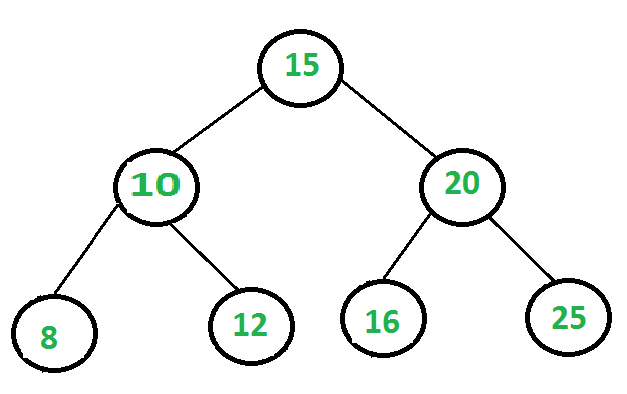GeeksforGeeks App
Open AppBrowser
Continue

# Print all internal nodes of a Binary tree

Given a Binary tree, the task is to print all the internal nodes in a tree.
An internal node is a node which carries at least one child or in other words, an internal node is not a leaf node. Here we intend to print all such internal nodes in level order. Consider the following Binary Tree:

Input:Output: 15 10 20

The way to solve this involves a BFS of the tree. The algorithm is as follows:

• Do a level order traversal by pushing nodes in the queue one by one.
• Pop the elements from the queue one by one and keep a track of following cases:
1. The node has a left child only.
2. The node has a right child only.
3. The node has both left and right child.
4. The node has no children at all.
• Except for case 4, print the data in the node for all the other 3 cases.

Below is the implementation of the above approach:

## C++

 `// C++ program to print all internal``// nodes in tree``#include ``using` `namespace` `std;` `// A node in the Binary tree``struct` `Node {``    ``int` `data;``    ``Node *left, *right;``    ``Node(``int` `data)``    ``{``       ``left = right = NULL;``       ``this``->data = data;``    ``}``};` `// Function to print all internal nodes``// in level order from left to right``void` `printInternalNodes(Node* root)``{``    ``// Using a queue for a level order traversal``    ``queue q;``    ``q.push(root);``    ``while` `(!q.empty()) {` `        ``// Check and pop the element in``        ``// the front of the queue``        ``Node* curr = q.front();``        ``q.pop();` `        ``// The variable flag keeps track of``        ``// whether a node is an internal node``        ``bool` `isInternal = 0;` `        ``// The node has a left child``        ``if` `(curr->left) {``            ``isInternal = 1;``            ``q.push(curr->left);``        ``}` `        ``// The node has a right child``        ``if` `(curr->right) {``            ``isInternal = 1;``            ``q.push(curr->right);``        ``}` `        ``// In case the node has either a left``        ``// or right child or both print the data``        ``if` `(isInternal)``            ``cout << curr->data << ``" "``;``    ``}``}` `// Driver program to build a sample tree``int` `main()``{``    ``Node* root = ``new` `Node(1);``    ``root->left = ``new` `Node(2);``    ``root->right = ``new` `Node(3);``    ``root->left->left = ``new` `Node(4);``    ``root->right->left = ``new` `Node(5);``    ``root->right->right = ``new` `Node(6);``    ``root->right->right->right = ``new` `Node(10);``    ``root->right->right->left = ``new` `Node(7);``    ``root->right->left->left = ``new` `Node(8);``    ``root->right->left->right = ``new` `Node(9);` `    ``// A call to the function``    ``printInternalNodes(root);` `    ``return` `0;``}`

## Java

 `// Java program to print all internal``// nodes in tree``import` `java.util.*;``class` `GfG``{` `// A node in the Binary tree``static` `class` `Node``{``    ``int` `data;``    ``Node left, right;``    ``Node(``int` `data)``    ``{``        ``left = right = ``null``;``        ``this``.data = data;``    ``}``}` `// Function to print all internal nodes``// in level order from left to right``static` `void` `printInternalNodes(Node root)``{``    ``// Using a queue for a level order traversal``    ``Queue q = ``new` `LinkedList();``    ``q.add(root);``    ``while` `(!q.isEmpty())``    ``{` `        ``// Check and pop the element in``        ``// the front of the queue``        ``Node curr = q.peek();``        ``q.remove();` `        ``// The variable flag keeps track of``        ``// whether a node is an internal node``        ``boolean` `isInternal = ``false``;` `        ``// The node has a left child``        ``if` `(curr.left != ``null``)``        ``{``            ``isInternal = ``true``;``            ``q.add(curr.left);``        ``}` `        ``// The node has a right child``        ``if` `(curr.right != ``null``)``        ``{``            ``isInternal = ``true``;``            ``q.add(curr.right);``        ``}` `        ``// In case the node has either a left``        ``// or right child or both print the data``        ``if` `(isInternal == ``true``)``            ``System.out.print(curr.data + ``" "``);``    ``}``}` `// Driver code``public` `static` `void` `main(String[] args)``{``    ``Node root = ``new` `Node(``1``);``    ``root.left = ``new` `Node(``2``);``    ``root.right = ``new` `Node(``3``);``    ``root.left.left = ``new` `Node(``4``);``    ``root.right.left = ``new` `Node(``5``);``    ``root.right.right = ``new` `Node(``6``);``    ``root.right.right.right = ``new` `Node(``10``);``    ``root.right.right.left = ``new` `Node(``7``);``    ``root.right.left.left = ``new` `Node(``8``);``    ``root.right.left.right = ``new` `Node(``9``);` `    ``// A call to the function``    ``printInternalNodes(root);``}``}` `// This code is contributed by``// Prerna Saini.`

## Python3

 `# Python3 program to print all internal``# nodes in tree` `# A node in the Binary tree``class` `new_Node:``    ` `    ``# Constructor to create a new_Node``    ``def` `__init__(``self``, data):``        ``self``.data ``=` `data``        ``self``.left ``=` `None``        ``self``.right ``=` `None``    ` `# Function to print all internal nodes``# in level order from left to right``def` `printInternalNodes(root):``    ` `    ``# Using a queue for a level order traversal``    ``q ``=` `[]``    ``q.append(root)``    ``while` `(``len``(q)):``        ` `        ``# Check and pop the element in``        ``# the front of the queue``        ``curr ``=` `q[``0``]``        ``q.pop(``0``)``        ` `        ``# The variable flag keeps track of``        ``# whether a node is an internal node``        ``isInternal ``=` `0``        ` `        ``# The node has a left child``        ``if` `(curr.left):``            ``isInternal ``=` `1``            ``q.append(curr.left)``        ` `        ``# The node has a right child``        ``if` `(curr.right):``            ``isInternal ``=` `1``            ``q.append(curr.right)``        ` `        ``# In case the node has either a left``        ``# or right child or both print the data``        ``if` `(isInternal):``            ``print``(curr.data, end ``=` `" "``)``    ` `# Driver Code``root ``=` `new_Node(``1``)``root.left ``=` `new_Node(``2``)``root.right ``=` `new_Node(``3``)``root.left.left ``=` `new_Node(``4``)``root.right.left ``=` `new_Node(``5``)``root.right.right ``=` `new_Node(``6``)``root.right.right.right ``=` `new_Node(``10``)``root.right.right.left ``=` `new_Node(``7``)``root.right.left.left ``=` `new_Node(``8``)``root.right.left.right ``=` `new_Node(``9``)` `# A call to the function``printInternalNodes(root)` `# This code is contributed by SHUBHAMSINGH10`

## C#

 `// C# program to print all internal``// nodes in tree``using` `System;``using` `System.Collections.Generic;` `class` `GFG``{` `// A node in the Binary tree``public` `class` `Node``{``    ``public` `int` `data;``    ``public` `Node left, right;``    ``public` `Node(``int` `data)``    ``{``        ``left = right = ``null``;``        ``this``.data = data;``    ``}``}` `// Function to print all internal nodes``// in level order from left to right``static` `void` `printInternalNodes(Node root)``{``    ``// Using a queue for a level order traversal``    ``Queue q = ``new` `Queue();``    ``q.Enqueue(root);``    ``while` `(q.Count != 0)``    ``{` `        ``// Check and pop the element in``        ``// the front of the queue``        ``Node curr = q.Peek();``        ``q.Dequeue();` `        ``// The variable flag keeps track of``        ``// whether a node is an internal node``        ``Boolean isInternal = ``false``;` `        ``// The node has a left child``        ``if` `(curr.left != ``null``)``        ``{``            ``isInternal = ``true``;``            ``q.Enqueue(curr.left);``        ``}` `        ``// The node has a right child``        ``if` `(curr.right != ``null``)``        ``{``            ``isInternal = ``true``;``            ``q.Enqueue(curr.right);``        ``}` `        ``// In case the node has either a left``        ``// or right child or both print the data``        ``if` `(isInternal == ``true``)``            ``Console.Write(curr.data + ``" "``);``    ``}``}` `// Driver code``public` `static` `void` `Main(String[] args)``{``    ``Node root = ``new` `Node(1);``    ``root.left = ``new` `Node(2);``    ``root.right = ``new` `Node(3);``    ``root.left.left = ``new` `Node(4);``    ``root.right.left = ``new` `Node(5);``    ``root.right.right = ``new` `Node(6);``    ``root.right.right.right = ``new` `Node(10);``    ``root.right.right.left = ``new` `Node(7);``    ``root.right.left.left = ``new` `Node(8);``    ``root.right.left.right = ``new` `Node(9);` `    ``// A call to the function``    ``printInternalNodes(root);``}``}` `// This code contributed by Rajput-Ji`

## Javascript

 ``

Output:

`1 2 3 5 6`

My Personal Notes arrow_drop_up